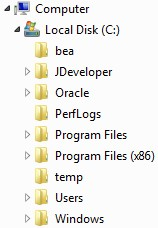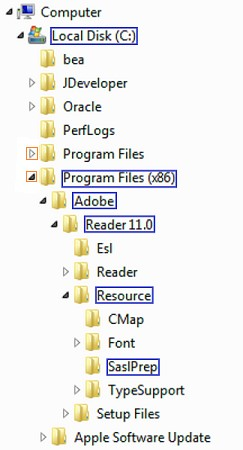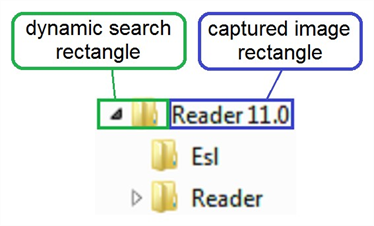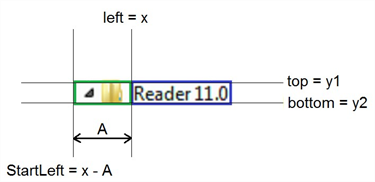# Dynamic Search Rectangles

Sometimes a GUI has numerous identical UI elements, but there is a requirement to interact with only certain instances of these elements. For example, in a file tree, it might be important to interact with specific nodes belonging to a particular folder while not interfering with other nodes in the tree.A possible testing scenario might be to navigate to folder C:\Program Files (x86)\Adobe\Reader 11.0\Resource\SaslPrep using the Windows Explorer file tree. Because the distance between the Folder name, folder icon, and node icon is often not static, it is important to limit the reference image to only the unique folder name. Even if this distance were static, capturing 2 images for each folder in the file path would be necessary: one for when the node is open and one when it is closed, so that the test doesn’t unintentionally open and close nodes.

The scenario would require 2 x 6 images, 12 images total, because the file path contains 6 folders.

## Example Use Case Scenario

Below is a strategy using dynamic search rectangles that can be used to create an efficient, reusable piece of code that opens folders according to an indicated file path. Because this strategy allows the capture of only 1 image of each folder and then an image of each of the two node states, the total number of images needed in our Suite is less, which reduces maintenance.

In this dynamic search rectangle strategy, only 6 + (2 x 1) images or 8 images total are necessary. The longer the file path and the more file paths in the test, the more effort that is saved on capturing images by using this method.

To begin, get familiar with the Points and Rectangles section of SenseTalk.

Below are some examples of using Rectangle functions with images.The image search will be based on the captured image rectangle (blue), but ultimately the element that the code interacts with is the dynamic icon (orange). The dynamic icon appears in the same position relative to the captured image rectangle.

put imageRectangle(FolderName) into myRectangle

set imageLeft = left (myRectangle)

set imageTop = top(myRectangle)

set StartLeft = imageLeft - 35

set UL = (StartLeft, imageTop)

set BR = (imageLeft, imageBottom)

set the SearchRectangle to (UL,BR)

In the above example, the SearchRectangle is set to (UL,BR) for all subsequent code (as a global property) until it is changed with additional code:

set the SearchRectangle to () -- set the SearchRectangle to full screen

Alternatively, the SearchRectangle can be set for a specific image search, as shown here:

If imageFound(ImageName:"NodeClosed", SearchRectangle:(UL,BR))

then

Do Action && foundImageLocation()

else if imageFound(ImageName:"NodeOpen", SearchRectangle:(UL, BR))

else

log "Highest Folder Level Reached."

end if

Here is a node-opening script called “Nodes” that makes use of both variables and parameters to make it a generic and reusable script:

Put imageRectangle("FirefoxIcon") into myRectangle

-- stores the upper left and lower right hand corner coordinates of the captured image rectangle into variable myRectangle

Set imageTop = top(myRectangle)

-- sets the variable "imageTop" as the y-coordinate of the upper left hand corner of variable myRectangle

Set imageRight = right(myRectangle)

-- sets the variable as the x-coordinate of the lower right hand corner

These functions can be used in scripts to create dynamic search rectangles based on one reference image.

1. Capture an image of each folder name (blue)
• “LocalDisk”
• “ProgramFilesx86”
• “Resource”
• “SaslPrep”
2. Capture an image of each node state (orange)
• “NodeOpen”
• “NodeClosed”In the following code, with A = 35 pixels, a SearchRectangle based on the captured image rectangle (blue) is set.

FolderName is a variable containing the name of the captured image rectangle (blue). Using variables such as FolderName will result in a more generic and therefore, a more reusable script.

//The code below opens the node for the initiated folder name if the node is not already open.

//Logs a message if a TargetFolder is found and if the highest folder level is reached.

params FolderName, Action, TargetFolder

If imageFound(FolderName)

then

put imageRectangle(FolderName) into myRectangle

set imageLeft = left(myRectangle)

set imageTop = top(myRectangle)

set imageBottom = bottom(myRectangle)

set StartLeft = ImageLeft - 35

set UL = (StartLeft, imageTop)

set BR = (imageLeft, imageBottom)

if imageFound(ImageName:"NodeClosed", SearchRectangle:(UL, BR))

then

Do Action && foundImageLocation()

else if imageFound(ImageName:"NodeOpen", SearchRectangle:(UL,BR))

then

else

log "Highest folder level reached"

end if

else

end if

if imagefound(folderName) and if TargetFolder

then

log "Target Folder" && FolderName && "Opened."

end if

Below is the master script that calls the node-opening script, Nodes, for each folder in a tree, starting with the first folder to be opened, LocalDisk. Note how the third parameter is optional.

Nodes "LocalDisk", Click

Nodes "ProgramFilesx86", Click